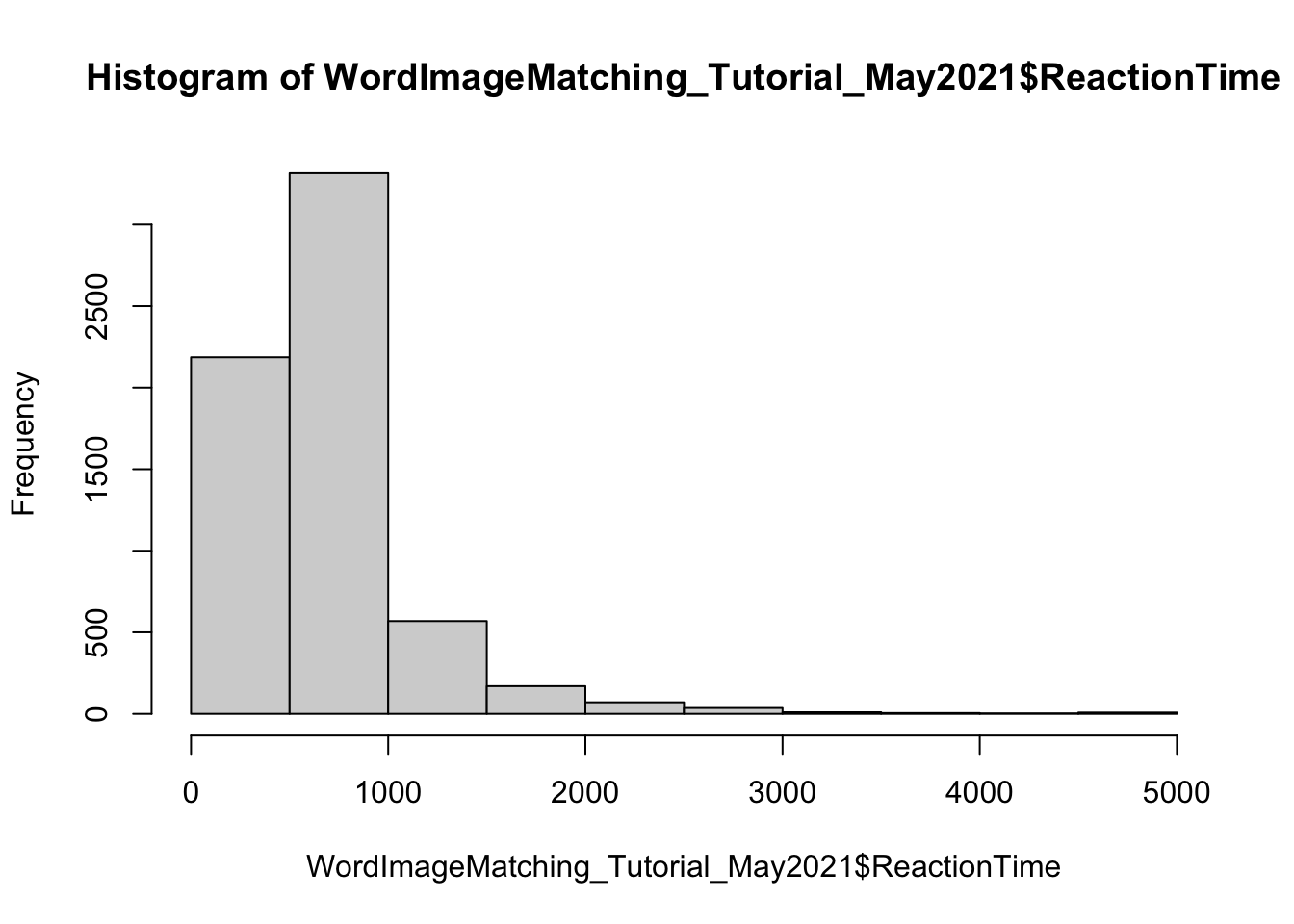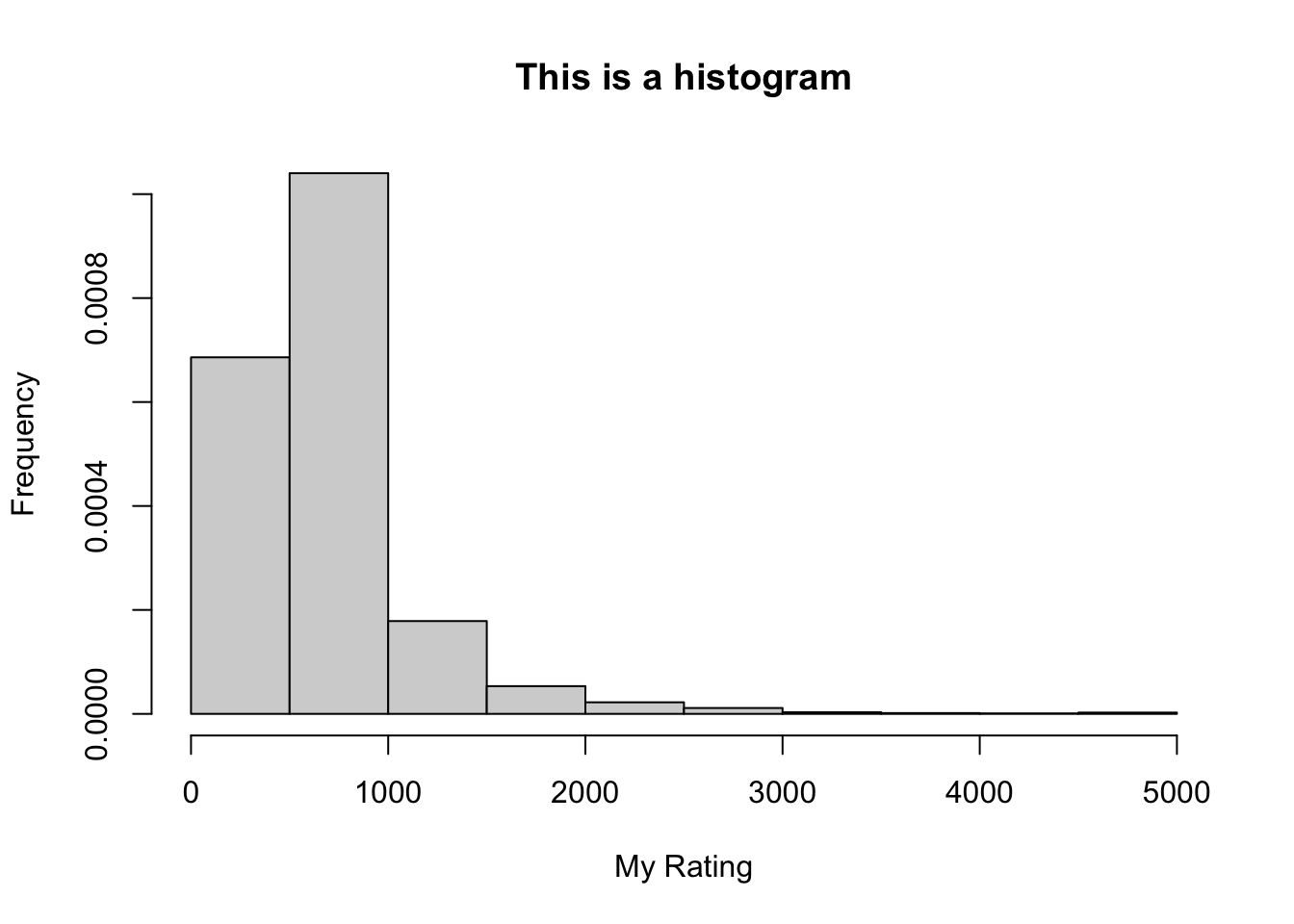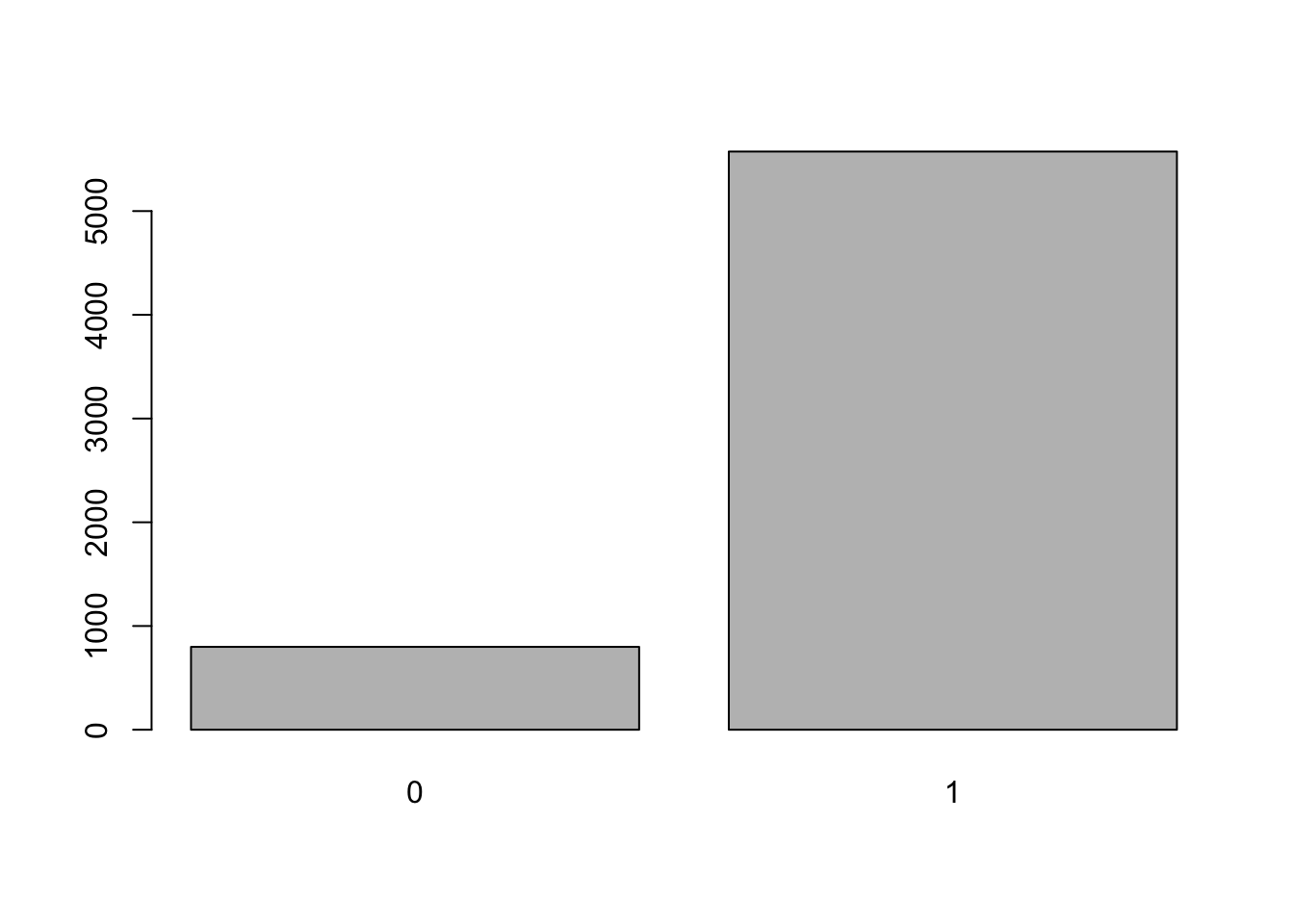# Session 3: Data Visualization

We will be usig some new data that contains reaction time and accuracy measures. This data file also contains item level information including language, lexical frequency and visual complexity, as well as participant level informaiton including language of the block (L1 or L2) and L2 usage. Please start by loading the “WordImageMatching_Tutorial_May2021.csv” file in to you R session.

Let’s also add in a chunk of code to ensure that our environment is clean, we are working in the correct directory and all required packages are installed and loaded.

``````#clean environment
remove(list = ls())

#check working directory
getwd() ``````
``##  "/Users/naominewmacbook/Desktop/Post-Doc I/R Tutorial"``
``````#load in data

#load packages (we won't need all of them today, but it's good practice to always begin by loading in some frequently used packages)
library(lme4)
library(lmerTest)
library(dplyr)
library(plyr)
library(ggplot2)
library(effects)
library(png)

#tell R not to use scientific notation
options(scipen=999)``````

R has some built in functions to create plots or you can use one of multiple different packages to make figures in R. The most commonly used and most well-documented one is ggplot2, which offers a wide variety of customizable plotting tools.

## 1. Base R Plots

If you are trying to take a quick look at your data to examine the distribution of a variable or look for outliers, you may easily do so using R’s built in plotting functions.

### 1.1. Histogram (continuous variable)

For example, you may use the hist() command to have R print out a histogram showing the distribution of the ReactionTime variable. Note that this will only work with numeric variables.

``````# most basic histogram
hist(WordImageMatching_Tutorial_May2021\$ReactionTime)              ````````````# slightly fancier histogram
hist(WordImageMatching_Tutorial_May2021\$ReactionTime,
xlab = "My Rating", ylab = "Frequency",        # Axes labels
main = "This is a histogram",                  # Plot title
freq = FALSE)                                  # Changes y axis from count (# of occurances) to frequency (percentage of occurances)``````### 1.2. Box Plot (binary or categorical variable)

For a quick way to look at how many observations in your data frame belong to each level of a categorical variable, you can use a bar plot.

``table(WordImageMatching_Tutorial_May2021\$Accuracy)   #this will calcualte the # of occurances per level``
``````##
##    0    1
##  799 5574``````
``barplot(table(WordImageMatching_Tutorial_May2021\$Accuracy))  #print a bar plot of this table``Exercise 1: Create a subset that only contains interlingual homograph trials, then examine the distribution of RT and accuracy

## 2. ggplot

ggplot2 is a powerful package that lets you plot anything from a simple scatter plot to an interactive 3D graph. It works by breaking up plots into semantic components such as scales and layers. This means that you can overlay multiple different plotting components over one another just by adding code. For example, you can make a scatter plot and then run a line through it or make a box plot showing means and CI and illustrate the individual data points as triangular shapes around the box.

Here’s a handy chart to help you decide which type of plot is most appropriate for your data: